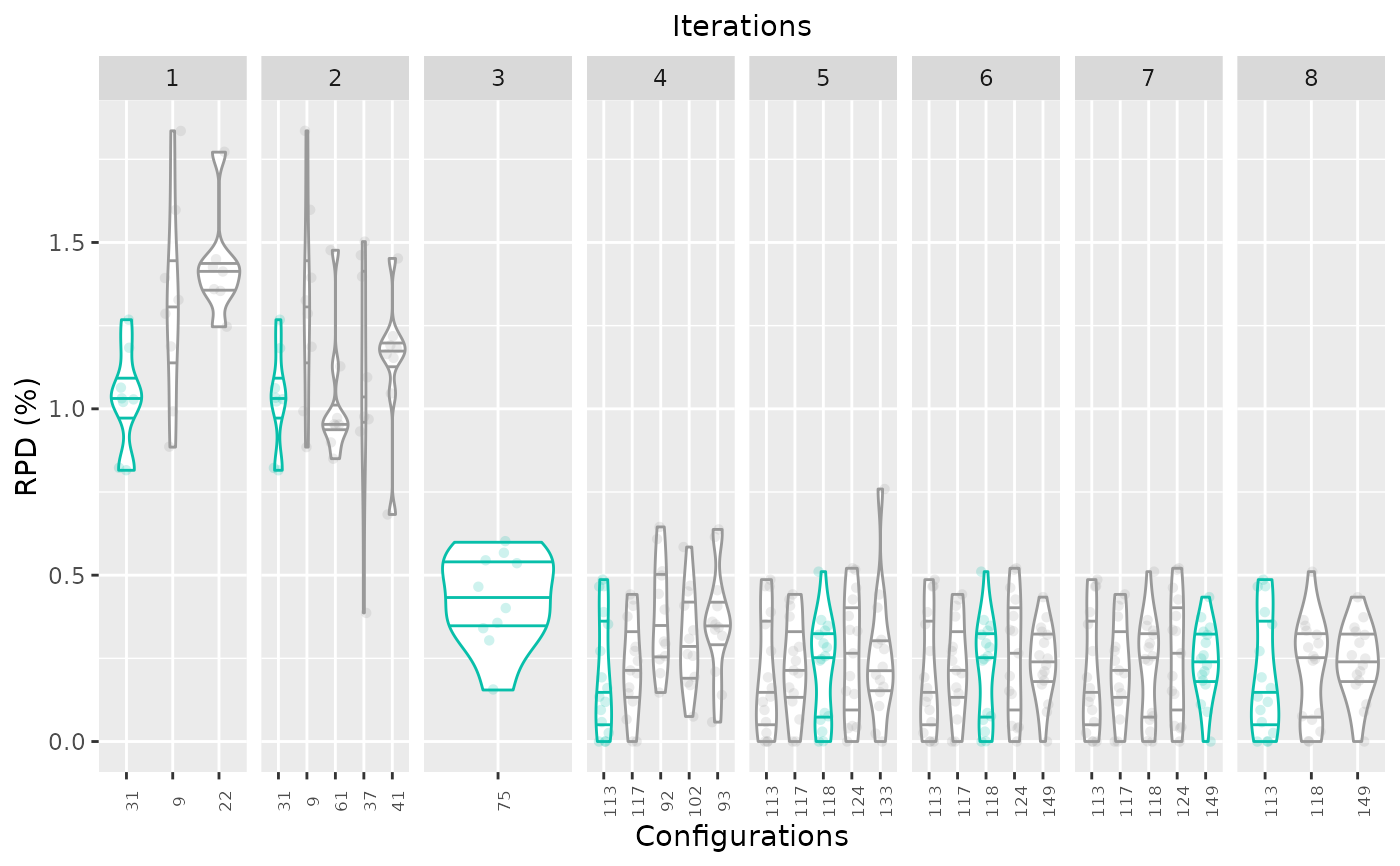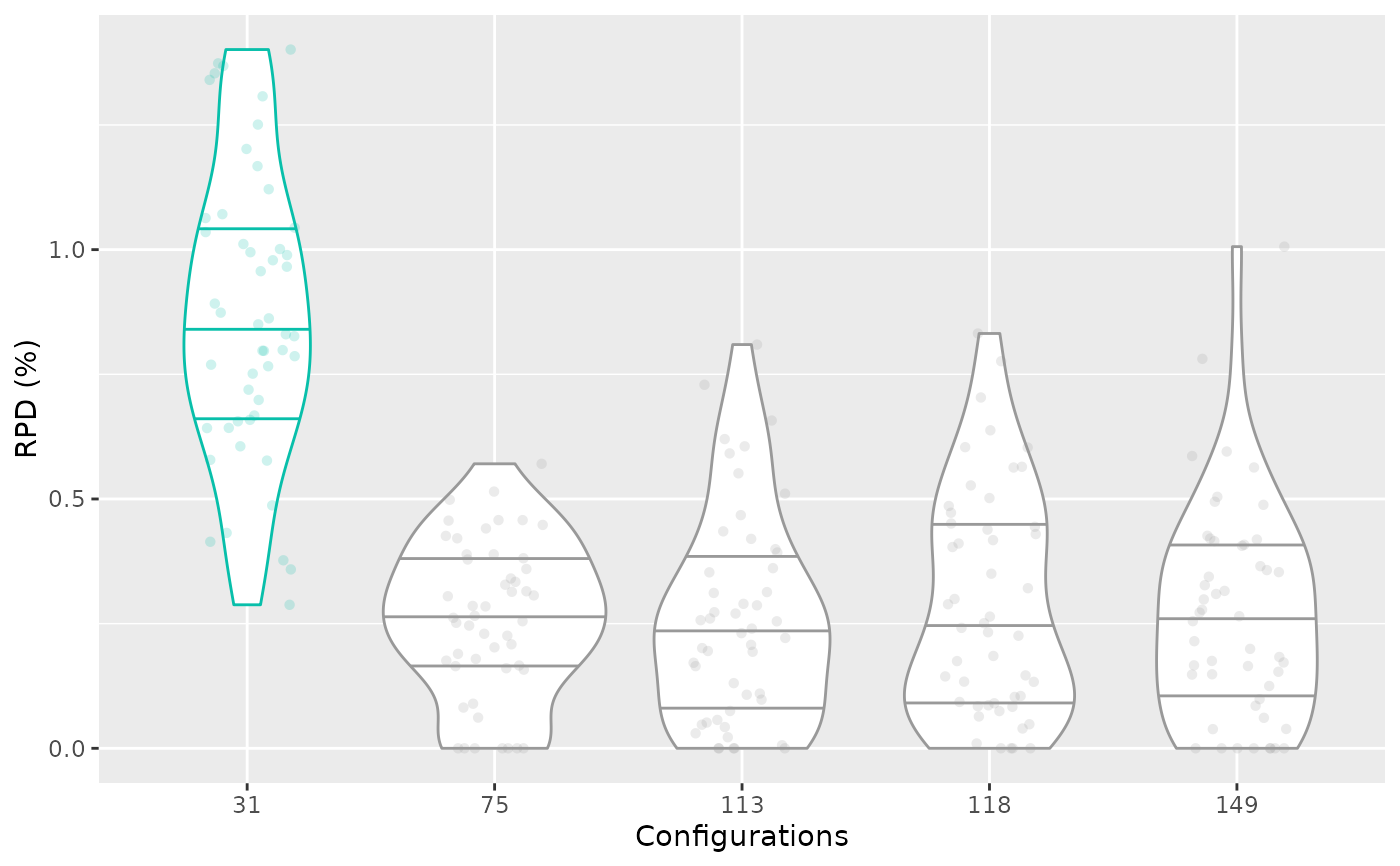Creates a box plot that displays the performance of a set of configurations which can be displayed by iteration.

boxplot_performance(
experiments,
allElites = NULL,
type = c("all", "ibest"),
first_is_best = TRUE,
rpd = TRUE,
show_points = TRUE,
best_color = "#08bfaa",
xlab = "Configurations",
boxplot = FALSE,
filename = NULL,
interactive = base::interactive()
)

## Arguments

experiments

Experiment matrix obtained from irace training or testing data. Configurations in columns and instances in rows. As in irace, column names (configurations ids) should be characters.

allElites

List or vector of configuration ids, (default NULL). These configurations should be included in the plot. If the argument is not provided all configurations in experiments are included. If allElites is a vector all configurations are assumed without iteration unless argument type="ibest" is provided, in which case each configuration is assumed to be from a different iteration. If allElites is a list, each element of the list is assumed as an iteration.

type

String, (default "all") possible values are "all" or "ibest". "all" shows all the selected configurations showing iterations if the information is provided. "ibest" shows the elite configurations of each iteration, note that the best configuration is always assumed to be first in the vector of each iteration.

first_is_best

Boolean (default TRUE) Enables the display in a different color the best configuration identified as the first one in a vector. If FALSE, all configurations are shown in the same color.

rpd

(logical(1)) TRUE to plot performance as the relative percentage deviation to best results per instance, FALSE to plot raw performance.

show_points

Logical, (default TRUE) TRUE to plot performance points together with the box plot.

best_color

String, (default "#08bfaa") color to display best configurations.

xlab

String, (default "Configurations") label for the x axis.

boxplot

By default, display a violin plot (ggplot2::geom_violin()). If TRUE, show a classical boxplot.

filename

(character(1)) File name to save the plot, for example "~/path/example/filename.png".

interactive

(logical(1)) TRUE if the report may use interactive features (using plotly::ggplotly(), plotly::plot_ly() and DT::renderDataTable()) or FALSE if such features must be disabled. Defaults to the value returned by interactive(),

## Value

ggplot2::ggplot() boxplot object

## Details

The performance data is obtained from the experiment matrix provided in the experiments argument. The configurations can be selected using the allElites argument and this argument can be also used to define the iteration of each elite configuration was evaluated.

boxplot_test() boxplot_training()

## Examples

iraceResults <- read_logfile(system.file(package="irace", "exdata",
"irace-acotsp.Rdata", mustWork = TRUE))
boxplot_performance(iraceResults$experiments, iraceResults$allElites)# \donttest{
boxplot_performance(iraceResults$testing$experiments, iraceResults\$iterationElites)# }